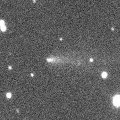# \$B%O%j%s%H%sWB@1(B

51P/Harrington (2008)###\$B###\$B50F;MWAG(B

```51P/Harrington
Epoch 2008 June 23.0 TT = JDT 2454640.5
T 2008 June 18.40250 TT                                 MPC
q   1.6877773            (2000.0)            P               Q
n   0.13830457     Peri.  269.17071     +0.98797346     +0.12273738
a   3.7032102      Node    83.77545     -0.07459664     +0.91108891
e   0.5442394      Incl.    5.42830     -0.13543921     +0.39351306
P   7.13
From 788 observations 1994 May 1-2009 Feb. 21, mean residual 1".1.
Nongravitational parameters A1 = +3.72, A2 = +0.0724.

51P-D/Harrington
Epoch 2008 June 23.0 TT = JDT 2454640.5
T 2008 June 17.98721 TT                                 MPCW
q   1.6876306            (2000.0)            P               Q
n   0.13833708     Peri.  269.17649     +0.98795833     +0.12283912
a   3.7026300      Node    83.76382     -0.07467718     +0.91107374
e   0.5442076      Incl.    5.42994     -0.13550517     +0.39351645
P   7.12
From 161 observations 1994 June 13-2015 May 30, mean residual 0".9.
Nongravitational parameters A1 = +5.67, A2 = +0.0667.
```

###\$B@1?^(B###\$B8wEYJQ2=(B

```        m1 =  6.5 + 5 log\$B&\$(B + 30.0 log r  [ ,0]  (              \$B!A(B2008\$BG/(B 6\$B7n(B18\$BF|(B)
m1 = 10.0 + 5 log\$B&\$(B + 15.0 log r  [0, ]  (2008\$BG/(B 6\$B7n(B18\$BF|!A(B              )

D\$B3K!'(B
m1 = 12.0 + 5 log\$B&\$(B + 30.0 log r  [ ,0]  (              \$B!A(B2008\$BG/(B 6\$B7n(B18\$BF|(B)
m1 = 15.5 + 5 log\$B&\$(B + 15.0 log r  [0, ]  (2008\$BG/(B 6\$B7n(B18\$BF|!A(B              )
```##### \$B50F;MWAG\$O(BM.P.E.C. 2012-J16\$B\$K7G:\\$5\$l\$?\$b\$N\$G\$9!#(B D\$B3K\$N50F;MWAG\$O(BM.P.E.C. 2015-L08\$B\$K7G:\\$5\$l\$?\$b\$N\$G\$9!#(B \$B@1?^\$O%9%F%i%J%S%2!<%?(B Ver.8 (\$B%"%9%H%m%"!<%D(B) \$B\$G:n@.\$7\$?\$b\$N\$G\$9!#(B \$B8wEY%0%i%U\$O(BComet for Windows\$B\$G:n@.\$7\$?\$b\$N\$G\$9!#(B